Contents >> Applied Mathematics >> Numerical Methods >> Numerical Integration >> The trapezoid rule

 Numerical integration - The trapezoid rule The trapezoid rule Let's consider the integral (2) representing, as is known, area under a curve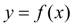on segment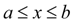(Fig. 1).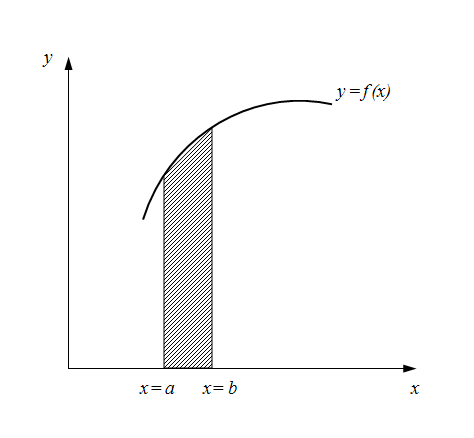Fig. 1. Geometrical representation of numerical integration Let's break now interval of integration  ( a , b )  to n equal parts in length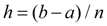everyone ( h is called step of integration ). We’ll consider one of these intervals (Fig. 2).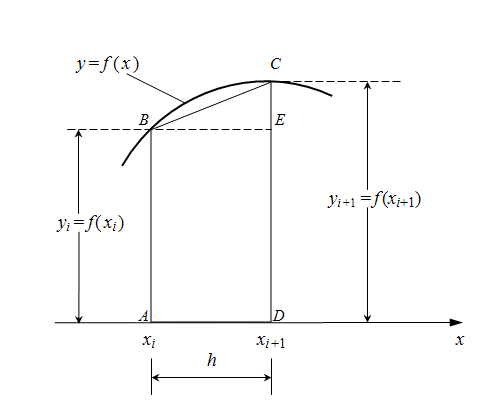Fig. 2. One interval of numerical integration by trapezoid method The area under a curvebetween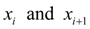is equal: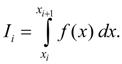Let's assume, that the step of integration h is small enough then this area without an essential error can be equated to the area of trapezoid ABCD . As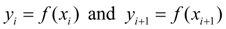, we’ll receive: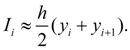(3) As integral from sum is equal to sum of integrals (property of additivity ), then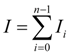,      (4) where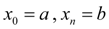. Substituting (3) in (4), we’ll finally receive [3, 4]: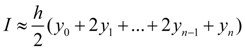.       (5) It also is the formula of trapezes . The trapezoid rule – one of elementary methods of numerical integration and though an error of calculations in this way has more, than in other methods, it is in great demand owing to its presentation and simplicity.

 < Previous Contents Next >